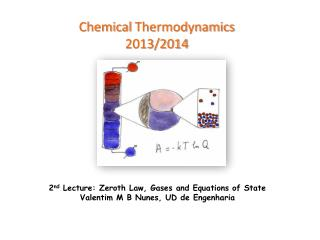DownloadDownload PresentationChemical Thermodynamics 2013/2014

# Chemical Thermodynamics 2013/2014

Download Presentation## Chemical Thermodynamics 2013/2014

- - - - - - - - - - - - - - - - - - - - - - - - - - - E N D - - - - - - - - - - - - - - - - - - - - - - - - - - -
##### Presentation Transcript

1. ChemicalThermodynamics2013/2014 2nd Lecture: Zeroth Law, Gases and Equations of State Valentim M B Nunes, UD de Engenharia

2. The Gaseous State When studying Thermodynamics we don’t need any theory about molecular structure but, in order to understand the subject, its good to have in mind molecular models for the states of matter. The gaseous state it is often taken as an example to describe the principles of Thermodynamics. In this Lecture we will review some of the basic concepts about the gaseous state, namely the concepts of pressure and temperature, and the basic laws of gases.

3. Ideal Gases A good vision of an ideal gas is an assembly of molecules, with sizes much smaller than average distances travelled between collisions, in continuous random (chaotic) movement, with velocities that increase with temperature, and that only interact in elastic collisions For a given system with volume, V, number of moles, n, and at pressure, p, and temperature, T, we say that it is at a given thermodynamic state. All this properties are not independent from each other. In other words they are related by an Equation of State. Before proceeding to those equations of state let us examine the concepts of pressure and temperature.

4. Pressure The pressure is a force exerted by an unit area. In the International System (SI) the unit for pressure is the Pascal (Pa). 1 Pa = 1 N.m-2 1 bar = 100 kPa (exact value) 1 atm = 101325 Pa (exact value) 1 atm = 760 mmHg (exact value)

5. Temperature Although the temperature is a fundamental physical concept, it is very difficult to define it. Temperature is the property that indicate us in what direction the heat flows! The definition of temperature results from the so called “zeroth law of thermodynamics”: if two systems are separately in thermal equilibrium with a third, then they must also be in thermal equilibrium with each other A, B and C share the same temperature.

6. Boyle´s Law At constant n and T, we obtain Isothermals of the gas.

7. Charles Law At constant n and p, we obtain Lord Kelvin was the first one to recognize the outstanding importance of this Law, giving origin to the absolute scale of temperatures. Isobaric of the gas

8. Avogadro's Law At constant pand T, we obtain

9. Perfect Gas Equation of State Combining the laws of gases we can easily obtain the perfect gas equation of state: Were R is the perfect gas constant. In the SI, R ≈ 8,314 J.K-1.mol-1. In non SI units it may be expressed by R ≈ 0,082 atm.L.K-1.mol-1 At STP (standard temperature and pressure), t=0 ºC and p=1 atm, the molar volume of a perfect gas, Vm = V/n is:

10. Surface of Possible States

11. pVT surface of an ideal gas

12. Mixtures: Dalton´s Law The pressure exerted by a mixture of perfect gases is the sum of the pressures exerted by the individual gases occupying the same volume Dalton´s Law writes as follow:

13. Real Gases For non-perfect gases there are present intermolecular forces, attractive and repulsive, between atoms and molecules, giving origin to deviations from ideality A quantitative measure o non-ideality is the compressibity factor, Z: Ideal gas Z = 1

14. Real Gas (CO2) pV isotherms Experimental isotherms for carbon dioxide CDE – vapor pressure of CO2 at 20 ºC The isotherm at 31,04 ºC corresponds to the critical isotherm * Critical point In the absence of intermolecular forces there will be no condensate states of matter (solids and liquids)!

15. Virial Equation of States At higher temperatures some of the isotherms are similar to the ideal gas. Perfect gas equation can be expanded, in terms of virial equations: Leiden form Berlin form B and B’ – 2nd virial coefficient C and C’ – 3rd virial coefficient

16. Virial coefficients: dependence of temperature

17. van der Waals equation van der Waals corrected the perfect gas equation by assuming that molecules occupy some space, and there are long–range intermolecular forces.

18. Molar volume calculation The VDW equation is cubic in volume, so it gives three roots for the volume in the VLE zone. Molar volume of liquid Molar volume of gas Without physical significance!

19. The critical isotherm For the critical isotherm there is an inflexion point. In mathematical terms we have: Solving these equations we have Solving altogether we obtain the critical values:

20. The Principle of Corresponding States If we define now a new set of parameters (reduced parameters): Substituting in the VDW equation we obtain: This means that two different gases at the same reduced temperature and reduced volume should exert the same reduced pressure, and they are in corresponding states.

21. Z factor for several gases

22. Equations of state